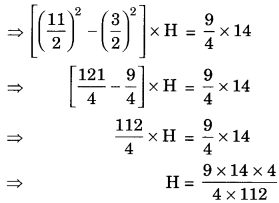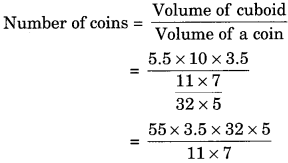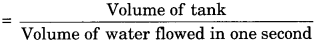# GSEB Solutions Class 10 Maths Chapter 13 Surface Areas and Volumes Ex 13.3

Gujarat Board GSEB Solutions Class 10 Maths Chapter 13 Surface Areas and Volumes Ex 13.3 Textbook Questions and Answers.

## Gujarat Board Textbook Solutions Class 10 Maths Chapter 13 Surface Areas and Volumes Ex 13.3

Question 1.
A metallic sphere of radius 4.2 cm is melted and recast into the shape of a cylinder of radius 6 cm. Find the height of the cylinder.
Solution:
In case of sphere, r = 4.2 cm
Volume of sphere = $$\frac {4}{3}$$πr3 = $$\frac {4}{3}$$π(4.2)3
In case of cylinder, r = 6 cm and h = ?
Volume of cylinder = πr3h = π x 63 x h
Volume of cylinder = Volume of sphere
⇒ π x 62 x h = $$\frac {4}{3}$$π (4.2)3
h = $$\frac{4 \times 4.2 \times 4.2 \times 4.2}{3 \times 6 \times 6}$$
h = 4 x 1.4 x 0.7 x 0.7
h = 2.74 cmQuestion 2.
Metallic spheres of radii 6 cm, 8 cm and 10 cm, respectively, are melted to form a single solid sphere. Find the radius of the resulting sphere.
Solution:
r1 = 6 cm
r2 = 8 cm
and r3 = 10 cm
Let radius of resulting sphere be r.
Volume of three spheres = Volume of resulting sphere
$$\frac {4}{3}$$πr31 + $$\frac {4}{3}$$πr32 + $$\frac {4}{3}$$πr33 = $$\frac {4}{3}$$πr3
$$\frac {4}{3}$$π [r31 + r32 + r33] = $$\frac {4}{3}$$πr3
⇒ r31 + r32 + r33 = r3
63 + 83 + 103 = r3
r3 = 216 + 512 + 1000
r3 = 1728
r = $$\sqrt{1728}$$
r = 12 cmQuestion 3.
A 20 m deep well with diameter 7 m is dug and the earth from digging is evenly spread out to form a platform 22 m by 14 m. Find the height of the platform. [NCERT 20121]
Solution:
In case of cylindrical well
Diameter = 7 m
r = $$\frac {7}{2}$$m
Depth of well h = 20 m
Volume of well or earth dug out = πr2h
= $$\frac {22}{7}$$ x $$\frac {7}{2}$$ x $$\frac {7}{2}$$ x 20 = 11 x 7 x 10 = 770 m3
In case of cuboidal platform
l = 22 m
b = 14 m
Let height of platform be h m.
Then volume of earth spread out in the form of cuboidal plate form = Volume of earth dug out from the well
l x b x h = 770
22 x 14 x h = 770
h = $$\frac{770}{22 \times 14}$$ = $$\frac{70}{2 \times 14}$$
h = 2.5 mQuestion 4.
A well of diameter 3 m is dug 14 m deep. The earth taken out of it has been spread evenly all around it in the shape of a circular ring of width 4 m to form an embankment. Find the height of the embankment. [CBSE 20121
Solution:
In case of cylindrical well
Diameter = 3 m
Radius r1 = $$\frac {3}{2}$$m
Depth h = 14m
Volume of earth dug out from well
= πr21h
= π ($$\frac {3}{2}$$)2 x 14
In case of circular embankment
= r + 4
= $$\frac {3}{2}$$ + 4 = $$\frac {11}{2}$$ m
Let height of embankment be H.
Volume of embankment = Volume of earth dug out
πR2H – πr2H = πr21h
π(R2 – r2)H = π($$\frac {3}{2}$$)2 x 14H = $$\frac {9}{8}$$
H = 1.125 m
Hence, the height of the embankment is 1.125 m.Question 5.
A container shaped like a right circular cylinder having a diameter 12 cm and a height 15 cm is full of ice cream. The ice cream is to be filled into cones of height 12 cm and diameter 6 cm, having a hemispherical shape on the top. Find the number of such cones which can be filled with ice cream.
Solution:
In case of right circular cylinder, diameter = 12 cm
r1 = 6 cm
h1 = 15 cm
Volume of ice cream in right circular cylinder
= πr12h1
= π x 62 x 15
In case of cone
Diameter = 6 cm
Height h2 = 12 cm
Volume of cone = $$\frac {1}{3}$$ πr22h2
= $$\frac {1}{3}$$ π x 32 x 12 = 36π cm3
Volume of hemisphere = $$\frac {2}{3}$$ πr3
= $$\frac {2}{3}$$π(3)3 = 187πcm3
Number of cones filled with ice creamQuestion 6.
How many silver coins, 1.75 cm in diameter and of thickness 2 mm, must be melted to form a cuboid of dimensions 5.5 cm x 10 cm x 3.5 cm?
Solution:
Silver coins
Diameter = 1.75 cm
Radius r1 = $$\frac {1.75}{2}$$ cm = $$\frac {1.75}{200}$$ = $$\frac {7}{8}$$cm
Thickness of coin h1 = 2 mm = $$\frac {2}{10}$$cm = $$\frac {1}{5}$$cm
Volume of a coin = πr21h1
= π x ($$\frac {7}{8}$$)2 x $$\frac {1}{5}$$
= $$\frac {22}{7}$$ x $$\frac {49}{64}$$ x $$\frac {1}{5}$$
= $$\frac{11 \times 7}{32 \times 5}$$ cm2
In case of cuboid
Length (l) = 5.5 cm
Height (h) = 3.5 cm
Volume of cuboid = l x b x h
= 5.5 x 10 x 3.5 cm3
= 192.5 cm3Hence, 400 silver coins must be method.Question 7.
A cylindrical bucket, 32 cm high and with radius of base 18 cm, is filled with sand. This bucket is emptied on the ground and a conical help of sand is formed. If the height of the conical heap is 24 cm, find the radius and slant height of the heap.
Solution:
In case of cylindrical bucket
Radius (r) of bucket = 18 cm
Height h = 32 cm
Volume of sand filled in cylindrical bucket
= πr2h
= π x 182 x 32
= 10368 π cm3
In case of conical heap
Height (H) = 24 cm
Volume of conical heap = Volume of cylindrical bucket
$$\frac {1}{3}$$πR2H = 10368π
$$\frac {1}{3}$$ x R2 x 24 = 10368
R2 = $$\frac{10368 \times 3}{24}$$ = 1296
R = $$\sqrt{1296}$$
R = 36 cm
Hence radius of conical heap of sand = 36 cm
I = $$\sqrt{\mathrm{R}^{2}+\mathrm{H}^{2}}$$ = $$\sqrt{36^{2}+24^{2}}$$
= $$\sqrt{1296+576}$$ = $$\sqrt{1872}$$ = 12 $$\sqrt{13}$$
Hence, slant height of sand = 12$$\sqrt{13}$$ cm.Question 8.
Water in a canal, 6 m wide and 1.5 m deep, is flowing with a speed of 10 km/h. How much area will it irrigate in 30 minutes, if 8 cm of standing water is needed?
Solution:
In case of canal
Width of canal (b) = 6 m
Depth of canal (h) = 1.5 m
Speed of flow of water = 10 km/h
= $$\frac{10 \times 1000 \mathrm{m}}{60 \mathrm{min}}$$ = $$\frac{500}{3}$$ m / minute
speed of water in 30 minute
= $$\frac{500}{3}$$ x 30 = 5000 m
Volume of water flowed in 30 minutes
= l x b x h
= 6 x $$\frac{3}{2}$$ x 5000 = 45000 m2
Volume of water stand in a field to irrigate = Volume of water flowed in 30 minutes
⇒ Area of field x $$\frac{8}{100}$$ = 45000
( ∴ height = 8 cm = $$\frac{8}{100}$$ m)
Area of field = $$\frac{45000 x 100}{8}$$ = 562500
1 hectare = 10000 m2
Area of field irrigate = $$\frac{562500}{10000}$$ = 56.25 hectaresQuestion 9.
A farmer connects a pipe of internal diameter 20 cm from a canal into a cylindrical tank in her field, which is 10 m in diameter and 2 m deep. If water flows through the pipe at the rate of 3 km/h, in how much time will the tank be filled?
Solution:
Diameter in case of cylindrical pipe = 20 cm
Radius (r) = 10 cm = $$\frac{10}{100}$$ m = 0.1 m
Speed of flow of water in pipe = 3 km / h
= $$\frac{3 \times 1000}{60 \times 60}$$ = $$\frac{5}{6}$$ m / s
Volume of water flowed per second
= πr2h = π(0.1)2 x $$\frac{5}{6}$$
In case of tank
Diameter = 10 m
R = 5m
Depth H = 2m
Volume of tank = πr2h
= π x 52 x 2 = 50π
Time taken to fill the tank= $$\frac{50 \pi}{\pi \times 0.1 \times 0.1 \times \frac{5}{6}}$$
= $$\frac{5000 \times 6}{1 \times 1 \times 5}$$
= $$\frac{6000}{60}$$ minutes = 100 minutes
= $$\frac{100}{60}$$ hour = 1 hour 40 minutes